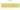# Fdj

## Djiboutian Franc

The official currency of Djibouti is the franc. DJF is its ISO 4217 currency code. It was once divided into 100 centimes.

#### how has the value of the currency changed in the last year?## USD 1 = DJF 178.66

How much is10 US dollars worth inDjiboutian francs?
At the current exchange rate, 10 US dollars is worth 1,786.55 Djiboutian francs
How much is50 US dollars worth inDjiboutian francs?
At the current exchange rate, 50 US dollars is worth 8,932.77 Djiboutian francs
How much is100 US dollars worth inDjiboutian francs?
At the current exchange rate, 100 US dollars is worth 17,865.53 Djiboutian francs
How much is500 US dollars worth inDjiboutian francs?
At the current exchange rate, 500 US dollars is worth 89,327.67 Djiboutian francs
How much is2,000 US dollars worth inDjiboutian francs?
At the current exchange rate, 2,000 US dollars is worth 357,310.68 Djiboutian francs
How much is10 US dollars worth inDjiboutian francs?
At the current exchange rate, 10 US dollars is worth 1,786.55 Djiboutian francs
How much is50 US dollars worth inDjiboutian francs?
At the current exchange rate, 50 US dollars is worth 8,932.77 Djiboutian francs
How much is100 US dollars worth inDjiboutian francs?
At the current exchange rate, 100 US dollars is worth 17,865.53 Djiboutian francs
How much is500 US dollars worth inDjiboutian francs?
At the current exchange rate, 500 US dollars is worth 89,327.67 Djiboutian francs
How much is2,000 US dollars worth inDjiboutian francs?
At the current exchange rate, 2,000 US dollars is worth 357,310.68 Djiboutian francs